Courses

# Alternative Current Practice Level - 1

## 35 Questions MCQ Test Exclusive Video Lectures of Class 12 Physics by Experts | Alternative Current Practice Level - 1

Description
This mock test of Alternative Current Practice Level - 1 for Class 12 helps you for every Class 12 entrance exam. This contains 35 Multiple Choice Questions for Class 12 Alternative Current Practice Level - 1 (mcq) to study with solutions a complete question bank. The solved questions answers in this Alternative Current Practice Level - 1 quiz give you a good mix of easy questions and tough questions. Class 12 students definitely take this Alternative Current Practice Level - 1 exercise for a better result in the exam. You can find other Alternative Current Practice Level - 1 extra questions, long questions & short questions for Class 12 on EduRev as well by searching above.
QUESTION: 1

Solution:
QUESTION: 2

Solution:
QUESTION: 3

### The figure shows a sine curve S(t) = sinωt and three other sinusoidal curves A(t), B(t) and C(t), each of the form sin (ωt -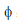). Which curve is according to the most negative value off.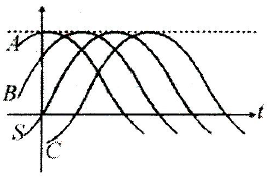Solution:
QUESTION: 4

A parallel-plate capacitor with rectangular plates is being discharged. A rectangular loop, centered on the plates and between them, measures L by 2L : the plates measure 2L by 4L. The fraction of the displacement current is encircled by the loop if that current is uniform

Solution:
QUESTION: 5

Figure shows three oscillating LC circuit with identical inductors and capacitors. If t1, t2, t3 are the time taken by the circuits I, II, III for fully discharge, then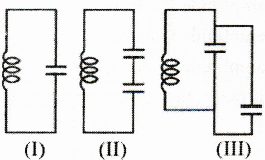Solution:
QUESTION: 6

In an oscillating LC circuit with L = 50 mH and C = 4.0 μF, the current is initially a maximum. How long will it take before the capacitor is fully discharged for the first time :

Solution:
QUESTION: 7

Charges on the capacitors in three oscillating LC circuits vary as follows (1) q = 2 cos 4t. (2) q = 4cos t, (3) q = 3 cos 4t, (4) q = 4 cos 2t, with q in coulomb and t in second. In which circuit (s) current amplitude is greatest:

Solution:
QUESTION: 8

A charged capacitor and an inductor are connected in series at time t = 0. Read the statements :

(1) the charge on the capacitor is zero after a time T/2

(2) The charge on the capacitor is zero after time T/4

(3) the energy stored in the capacitor maximum at T/2

(4) the energy stored in the inductor is maximum at T/4

Solution:
QUESTION: 9

If we increase the driving frequency in a circuit with a purely capacitive load. Read the following statements
(1) amplitude Vc increases
(2) amplitude Vc decrease
(3) amplitude ic increase
(4) amplitude ic decreases
Out of these, the correct statement(s) is/are

Solution:
QUESTION: 10

If we increase the deriving frequency in a circuit with a purely inductive load. Read the following statements :
(1) amplitude VL remain constant
(2) amplitude VL increases
(3) amplitude iL increases
(4) amplitude iL decreases
Out of these, the correct statement (s) is / are

Solution:
QUESTION: 11

Here are the capacitive reactance and inductive reactance, respectively, for three sinusoidally driven
RLC circuits : (1) 50 W, 100 W (2) 100 W, 50 W (3) 100 W,100 W
Which is in resonance ?

Solution:
QUESTION: 12

An alternating current emf device has a smaller resistance than that of the resistive load, to increase the transfer of energy from the device to the load, a transformer will be connected between two. Then

Solution:
QUESTION: 13

The figure here gives the electric field of an electromagnetic wave at a certain point and a certain instant. The wave is transporting energy in the negative z-direction. The direction of the magnetic field of the wave at that point and instant is :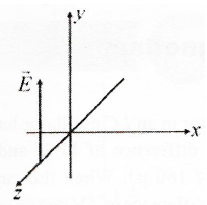Solution:
QUESTION: 14

Which of the following plots may represent the eactance of a series LC combination ?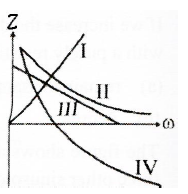Solution:
QUESTION: 15

An AC source is rated 220 V, 50 Hz. The average voltage is calculated in a time interval of 0.01s. It

Solution:
QUESTION: 16

An electromagnetic wave going through vacum is described by E = E0 sin (kx-ωt) ; B = B0 sin (kx-ωt) .
Then

Solution:
QUESTION: 17

Alternating current can not be measured by dc ammeterbecause

Solution:
QUESTION: 18

If a current I given by I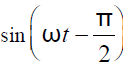flows in an ac circuit across which an ac potential of V = V0 sinωt has been applied, then the power consumption P in the circuit will be

Solution:
QUESTION: 19

An alternating voltage V = 200√2 sin(100t) is connected to a 1 microfarad capacitor through an ac ammeter. The reading of the ammeter shall be

Solution:
QUESTION: 20

A 220 V, 50 Hz ac source is connected to an inductance of 0.2 H and a resistance of 20 ohm in series. What is the current in the circuit?

Solution:
QUESTION: 21

In the circuit shown below, the ac source has voltage V = 20 cos(ωt ) volt with ω = 2000 rad/s. the amplitude of the current will be nearest to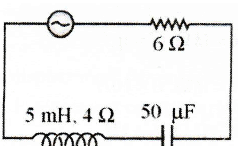Solution:
QUESTION: 22

The power factor of an ac circuit having resistance (R) and inductance (L) connected in series and an angular velocity ω is

Solution:
QUESTION: 23

An inductor of inductance L and resistor of resistance R are joined in series and connected by a source of frequency ω . Power dissipated in the circuit is

Solution:
QUESTION: 24

In a LCR circuit capacitance is changed from C to 2C. For the resonant frequency to remain unchanged, the nductance should be change from L to

Solution:
QUESTION: 25

In an LCR series ac circuit, the voltage across each of the components, L, C and R is 50 V. The voltage across the LC combination will be

Solution:
QUESTION: 26

A bulb and a capacitor are connected in series to a source of alternating current. If its frequency is increased, while keeping the voltage of the source constant, then

Solution:
QUESTION: 27

An alternating e.m.f. of angular frequency ω is applied across an inductance. The instantaneous power developed in the circuit has an angular frequency

Solution:
QUESTION: 28

The diagram shows a capacitor C and a resistor R connected in series to an ac source. V1 and V2 are voltmeters and A is an ammeter.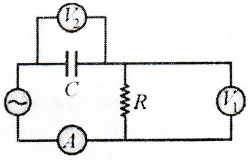Consider now the following statements
I. Readings in A and V2 are always in phase
III. Readings in A and V1 are always in phass.
Which of these statements are/is correct?

Solution:
QUESTION: 29

The voltage of an ac supply varies with time (t) as V = 120 sin100πtcos100 πt . The maximum voltage and frequency respectively are

Solution:
QUESTION: 30

Match the following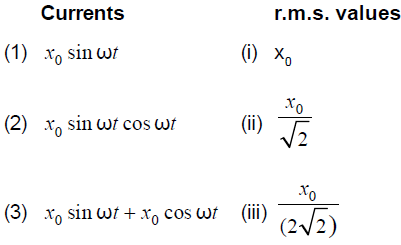Solution:
QUESTION: 31

An ac source of angular frequency ω is fed across a resistor r and a capacitor C in series. The current registered is I. If now the frequency of source is changed to ω / 3 (but maintaining the same voltage), the current in the circuit is found to be halved. Calculate the ratio of reactance to resistance at the original frequency ω

Solution:
QUESTION: 32

The output current versus time curve of a rectifier is shown in the figure. The average value of output current in this case is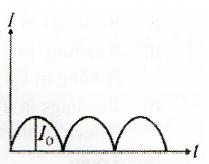Solution:
QUESTION: 33

When an ac source of e.m.f. e = V0 sin(100t) is connected across a circuit, the phase difference between the e.m.f. e and the current i in the circuit is observed to be π / 4, as shown in the diagram If the circuit consists possibly only of RC or LC in series, find the relationship between the two elements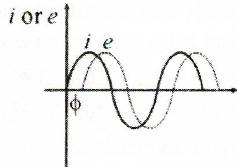Solution:
QUESTION: 34

In an oscillatory circuit, L = 0.4 H, C = 0.0024 μF. Themaximum value of the resistance that should be included is:

Solution:
QUESTION: 35

At t < 0, the capacitor is charged and the switch is opened. At t = 0 the switch is closed. The shortest time T at which the charge on the capacitor will be zero is given by :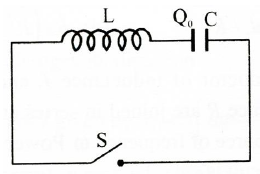Solution: